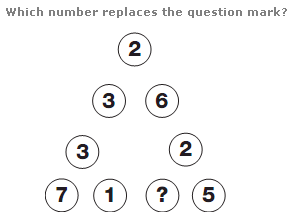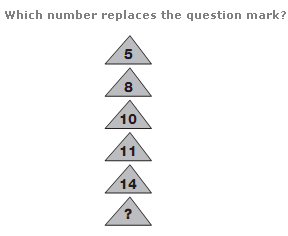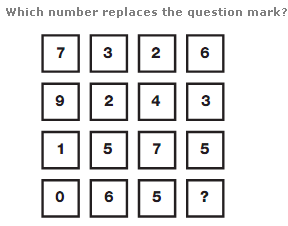Puzzles - Number puzzles

Exercise :: Number puzzlesAnswer : 2 Explanation : The sum of the numbers along each side of the triangle is always 15.Answer : 16 Explanation : As you move downwards, numbers increase by 3, then 2, then 1, before repeating this pattern.Answer : 7 Explanation : The numbers in each row of the diagram add up to 18.